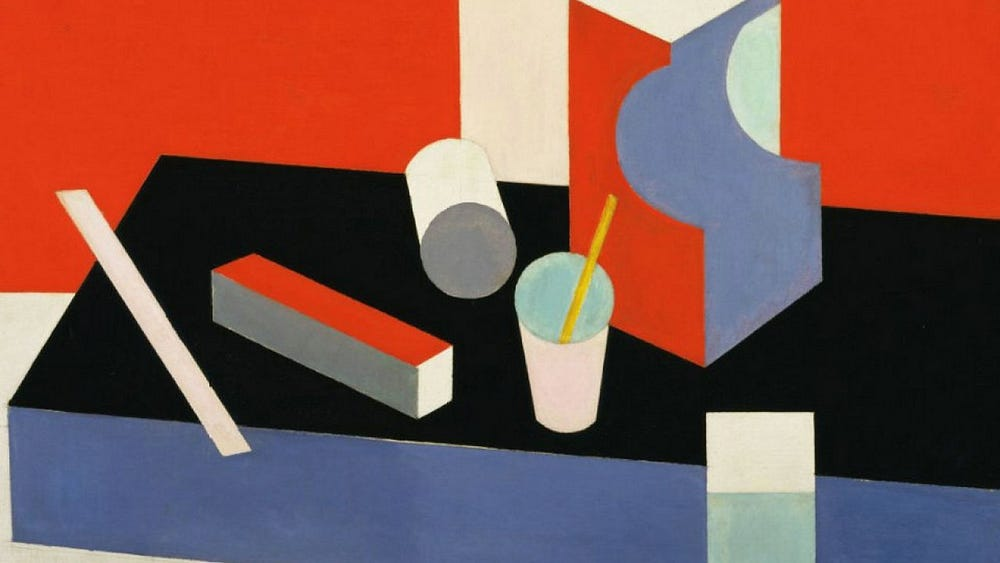# Creating an Art Period Classifier with Create ML

Original article was published on Artificial Intelligence on Medium# What exactly is a Classifier?

A classifier is an algorithm that sorts data into labeled classes, or categories, of information. There are many examples of classifiers out there, but the best one is probably an email spam filter. They are good examples of pattern recognition in machine learning (I would read this article to learn more about Machine Learning). To sum up what machine learning is, it is a system that allows computers to learn automatically without human intervention. They work by taking data, finding the pattern, and applying the pattern. A good example of this is email spam classifiers. These classifiers look through emails and classify them as spam or not as spam. Classifiers are super useful as they make the most practical use of machine learning.

Classifiers are also usually put into two different categories: supervised learning and unsupervised learning. I already wrote an article that gives a more detailed explanation on how the difference between supervised learning and unsupervised learning when I built my image classifier(Click Here to read). To summarize, the main difference comes from the training data. Supervised learning uses labeled data (which I used for this product) and learns to create an algorithm and make predictions. The goal of Supervised learning is to create an algorithm that can accurately predict something based on the given data. Unsupervised learning is when the model is given unlabeled data and it has to find a pattern to come up with an algorithm. The goal of unsupervised learning is to find the pattern within the data.

There are also different ways that classifiers classify images. Here are a few methods of how it does that:

## Decision Tree

Have you ever made a factor tree when you were little? A decision tree looks like that. They are sort of like a flowchart. You get a question and depending on what you answer you follow to the next question, and you repeat until you reach a final decision. Classifiers follow this same thing. They set up these if-then rules to follow to create ways to classify the data.

## Naive Bayes

Naive Bayes is a classifier that’s inspired by Bayes theorem. Bayes’ theorem describes the probability that an event based on pasta data. For example, if the risk of health problems increases as you age, Bayes’s theorem can guess the risk of an individual based on their age, rather than what the entire population is suffering from.

## Artificial Neural Networks

Artificial Neural Networks are a set of input and output layers that work to classify the data. Each layer contains nodes that have different weights and biases to help classify the data better. These networks usually have multiple hidden layers that help classify the data.

## K-Nearest Neighbor

This is known as a lazy learning algorithm that works on analyzing unknown places by classifying the nearest data-points. For example, if there is an unknown data point, it looks at other know data-points near it to decide the best possible answer.